×

×

UF / Statistics / STA 3032 / A statistical hypothesis is the claim that some population characteris

# A statistical hypothesis is the claim that some population characteris Description

##### Description: These notes cover the basic outline of Modules 3-4.3 as covered by the professor. Module 3 is very similar to the weekly notes. This is just a basic outline of what will be on the exam excluding contingency tables because he has not yet covered those!
9 Pages 90 Views 5 Unlocks
Reviews

Jarred Bartell (Rating: )

I love that I can count on (Tia for top notch notes! Especially around test time...

MODULE 3

## A statistical hypothesis is the claim that some population characteristic is equal to what?ARTICLE I.

3.1 INFERENCE FOR ONE POPULATION

1) When a population parameter is estimated by a sample statistic, this sample estimate is not  100% representative of the population because it varies from sample to sample.

3.1.1 CONFIDENCE INTERVALS

1) Confidence Interval (CI): reports an interval of plausible values based on the point estimate  sample statistic and its standard deviation

a. To calculate a confidence interval you first

i. Select the confidence level 100(1-α)%

ii. If the sample is replicated many times, the proportion of times that the CI will  not contain the population parameter is α

2) Known population variance

## What are the error types in testing the hypothesis?a. Assume you have µ and σ2

i. The methodology to calculate a CI will require a normal distribution such that  X  ~ N(μ,
) for  > 30

ii. If n is less than 30 then we will use t values instead of Z *will be elaborated on  later in notes*

iii. Note that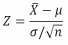## How to know the population variances?If you want to learn more check out In microeconomics, what is the method of marginal decision­ making?

b. If the data is normally distributed, you calculate CI using Z scores

i. The probability that the CI interval-

-contains the true value of µ is 1-α.

ii. In most cases CI will be constructed from a single sample and we can no longer  talk about probability

1. But we can say that we are 100(1-α)% confident that the methodology

by which the interval was contrived will contain the true population

parameter (most of the time µ)

3) Unknown population variance

a. When a sample size is large we can assume that s2 is representative of σ2

b. When you don’t know the population variance (σ2), you use the t-test

i. The t-test is determining the confidence interval using a t value instead of z

ii. Note that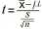*where t= tn-1  If you want to learn more check out What is elastic cartilage?

iii. The n-1 is for degrees of freedom (v=n-1) which is used when finding the

necessary value on the t-table

3.1.2 HYPOTHESIS TESTS

1) A statistical hypothesis is the claim that some population characteristic is equal to a certain  value  If you want to learn more check out What is gonadal sex determined by?

a. The null hypothesis (denoted by H0) is the hypothesis that is initially assumed to be true  b. The alternate hypothesis (denoted by H1) is the complementary to H0, usually the  hypothesis that is wished to be tested.  We also discuss several other topics like What does long run aggregate supply represent?

2) Test procedure

a. Created under the assumption of the null and then it is determined how likely that  assumption is compared to the alternate

i. Test statistic- a function of the sampled data

ii. Rejection region/criteria- set of all test statistic values for which the null will be  rejected

b. Error types

i. Type I error consists of rejecting the null when it is in fact true

ii. Type II error consists of failing to reject the null when it is in fact false

3) Known population variance

a. A test statistic is an unbiased estimator of a population parameter.

b. The test statistic correspondent to the t or z value depending on the information given.  This test statistic will give you a value in which you need to find a p-value from either  your z-table or t-table.

4) P Value  We also discuss several other topics like What are the components of aggregate expenditures?

a. The p-value of a hypothesis test is the probability of observing the specific value of the  test statistic, or a more extreme value, under the null hypothesis.

b. The criteria for rejecting the null is p-value is less than α (generally less than 0.05)  5) Unknown population variance

a. If σ is unknown, which is common, we replace it with s

b. At the α significance level we will still reject the null if the p value is less than α  i. Note: the values within a two sided 100(1-α)% will result in the p-value to be  greater than α

c. Note that: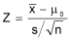and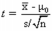3.2 INFERENCE FOR POPULATION PROPORTION

3.2.1 LARGE SAMPLE CONFIDENCE INTERVAL

1) The classical way of handling large sample binary statistics was using the CLT which tells us that  for X~Bin(n,p)  If you want to learn more check out What were luther's ideas?

a. p̂=x/n

b.  ̂~    ,  (   )

c. A 100(1-α)% CI can be created as  ̂ ±     /    (    )

2) However this is not applicable to small sample sizes. Therefore we use the Agresti Coull wehre a  100(1-α)% CI is: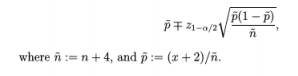3.2.2 LARGE SAMPLE HYPOTHESIS TEST

1) Let’s say that X~Bin(n,p), then by the CLT

̂~    , (   )

(when the number of successes and failures is greater than 5)

2) To test the null hypothesis for H0: p≤p0 or p≥p0 or p=p0,

a.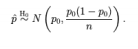we must assume that np0>5 and n(1-p0)>5

b. The test statistic would be: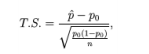Where you reject the null if p-value< α

3.3 INFERENCE FOR POPULATION VARIANCE

1) We can calculate the estimated population variance with

2) also note that this is true: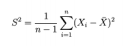*where X2 is representative of the chi square distribution with n-1 degrees of freedom3.3.1 CONFIDENCE INTERVAL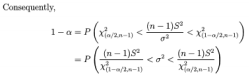3.3.2 HYPOTHESIS TEST

1) To test something dealing with σ2 we use test statistic: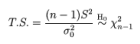Where the null hypothesis is still rejected when p-value<α  3.4 DISTRIBUTION FREE INTERFERENCE

1) When using small sample sizes:

a. We cannot assume normally distributed data

b. We need to use exact nonparametric procedures when finding statistics

c. Instead of means we will use medians because they are less influenced by outliers  3.4.1 SIGN TEST

1) Recall that a pth percentile includes all data that falls above (1-p)%

a. Let B denote the number of observations greater than the pth percentile

2) B~Bin(n,1-p) where µp denotes the population pth percentile

3) We can test hypotheses dealing with µp

a. The p-value must still be smaller than α to reject the null hypothesis

3.4.2 WILCOXON SIGNED-RANK TEST

1) In this case, the null hypothesis is that the distribution of data is centrally located around a  certain value µ0. This value is tested against X.

a. The test determines whether Xs tend to be larger, smaller, or different than µ0.  2) To carry out the test:

a. Center the data according to the null hypothesis by calculating the differences between  your data values (X) and µ0

b. Rank the absolute values of the differences

c. Calculate the test statistic, s+, by adding all off differences

3) Note:

4) The test statistic, denoted by W: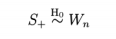MODULE 4

ARTICLE II.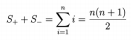INFERENCE FOR POPULATION MEANS

1) Confidence Intervals

a. We can find confidence intervals of two difference sample means

2) Known Population Variances

a. Let X and Y be to independent binomial random variables.

b. A 100(1-α)% CI for the difference of the two means from each random variable is: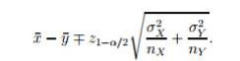3) Unknown population variance

a. t-value is used due to estimation of variance

b. therefore, we need degrees of freedom (V) so we use the equation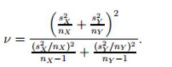c. The Confidence interval with t value and estimated variance would be:

4) Large sample confidence intervals for two population proportions

a. Let X and Y be to independent binomial random variables.

b. Proportion p is the number of successes/n

c. Let ñ̃x=nx+2

d. Let p̃x=(x+1)/ ñ̃x

e. The CI is therefore:

Note: the difference of two proportions should lie between -1 and 1

5) Paired Data

a. There are instances when two samples are not independent, this is when the data is  paired.

b. It is referred to as paired because we consider the data in pairs, (X1,Y1)

c. We use the data set of differences, D1=X1-Y1 for calculation

i. This reduces a 2 sample problem to a 1 sample problem

d. This holds equivalently for the means of two separate samples

e. NOTE: differences (D) can be incorporated into covariance by:

4.1.2 HYPOTHESIS TESTS

1) Known variance

a. Let Xn and Yn represent two independent random large samples

i. Note: to be large nx>40 and ny>40

ii. We can assume XM and YM are normally distributed and therefore:

b. The null hypothesis would be in the form of µx-µy is

i. less than or equal to

ii. greater than or equal to

iii. or equal to ∆!

iv. i.e.

c. Test statistic

i. It still holds true that if the p-value from the test statistic formula is less than α  then we reject the null hypothesis

2) Unknown variance test for difference of two means

a. Usually if the variances are unknown then they have to be estimated (s2 instead of σ2)  and the test statistic is

b. The degrees of freedom are given by the previous equation:

3) Large sample test for two population proportions

a. Let

b. The null hypothesis will be similar to the previous one except instead of µx-µy it will be  px-py

i. i.e.

4) We assume the number of successes and failures is greater than 10 for both samples  5) The test statistic would be

NOTE: when ∆! = zero, it is assumed the proportions are equal which implies the two variances  are equal and therefore we can use the new test statistic of:

6) Paired data

a. In the event that the two samples are dependent, we use the data of the differences of  X and Y

b. Hence the test statistic would be

4.2 INFERENCE FOR POPULATION VARIANCES

1) Now we deal with two independent normal distribution samples

a. We can infer that:

2) F-distribution

a. It is known that a standardized ratio of the two X2’s is an F-distribution

i. An F-distribution is said to be a continuous probability distribution. It is

technically the null distribution of a test statistic and it analyzes variance.

b. Therefore:

4.2.1 CONFIDENCE INTERVALS OF F-DISTRIBUTIONS

1) F-distribution

2) A 100(1-α)% confidence interval for "# /"\$

NOTE: if creating a one-sided C.I. simply replace α/2 with α. If creating an upper, replace  lower limit with 0 and if creating a lower, replace the upper limit with infinity.

4.2.2 HYPOTHESIS TESTS

1) Instead of testing µx-µy or px-py, we are testing "# /"\$

a. i.e.

2) The test statistic is:

NOTE: the null hypothesis is not necessarily rejected when the p-value<α

4.3 DISTRIBUTION FREE INFERENCE

4.3.1 WILCOXON-RANK SUM TEST

1) Used for the differences between two samples. It tests whether:

a. Y’s tend to be larger than the X’s

b. Y’s tend to be smaller than the X’s

c. One of the two populations is shifted from the other

2) To conduct the test we:

a. First rank all the (nx+ny) data irrespective of the sample

b. Then calculate the sum of the ranks associated with the smallest sample

c. We use this test statistic to find the p-value

4.3.2 WILCOXON SIGNED-RANK TEST

1) Used for the differences between two samples. In this case we take the differences (D’s) and  test the null hypothesis

a. H0 : Distribution of D’s is symmetric about the null value ∆! against the alternatives of D  values tending to be smaller than ∆! or D values tending to be larger than ∆!

b. Rank the absolute values of the differences

c. Calculate the test statistic, s+, by adding all off differences

2) Note:

3) The test statistic, denoted by W:

4) The null is rejected if

Page ExpiredIt looks like your free minutes have expired! Lucky for you we have all the content you need, just sign up here